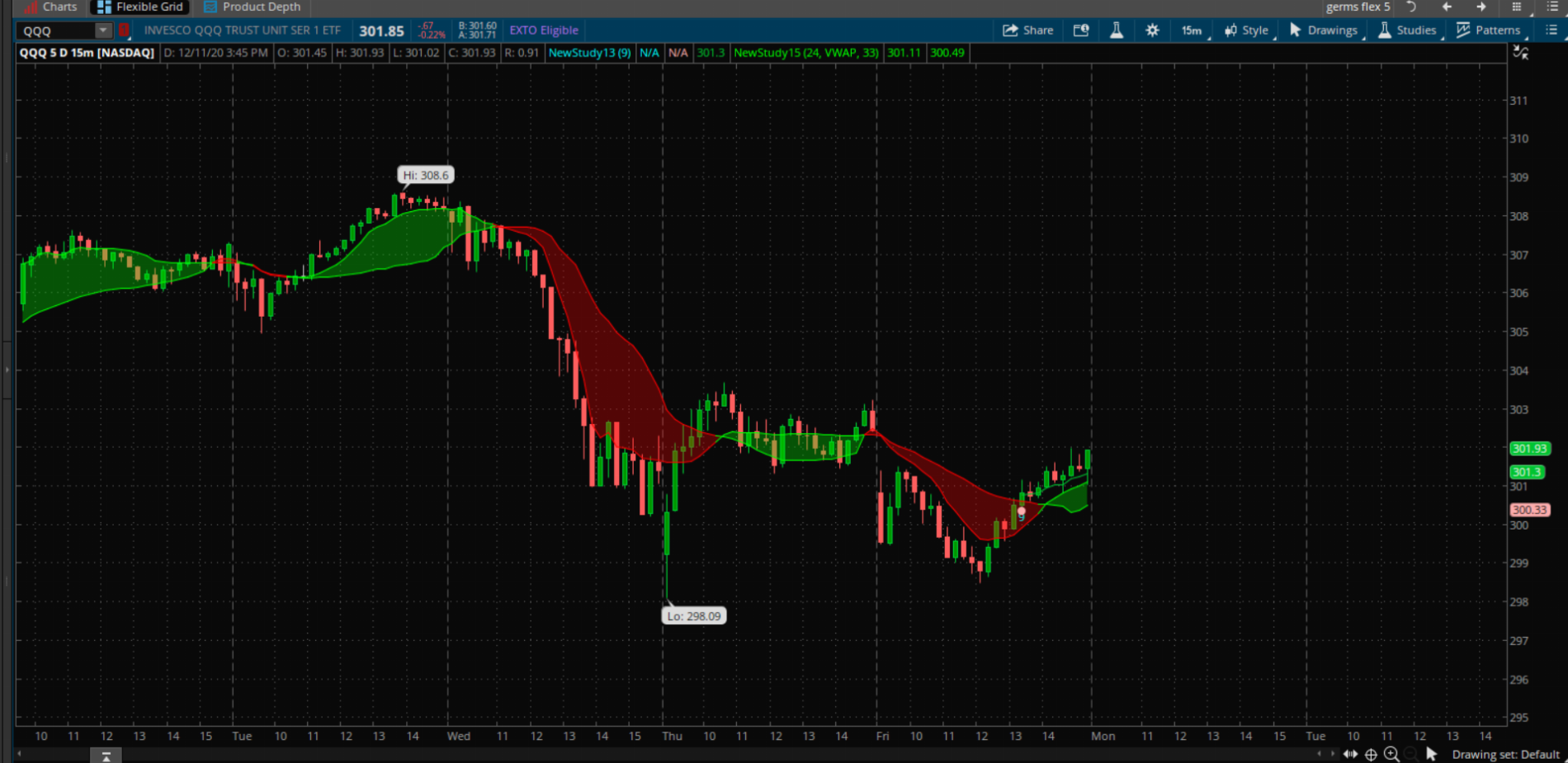# Squeeze Clouds based on SMA and VWAP

#### germanburrito

##### Active member
These clouds consist of a deviation scaled sma, as well as a vwap scaled sma, these clouds may act as support and resistance because of the nature of being weighted by volume and standard deviation of set period, when the bands squeeze it may indicate a reversal or a weakening trend, when the bands expand the opposite is true.Code:
``````# Nube 6.24.19

# German_burrito

input length = 24;

input price  = vwap;
input length2 = 34;

def p = if IsNaN(price) then p else price;
def v = if IsNaN(volume) then v else volume;
def zeros = close - close;
def filter = reference EhlersSuperSmootherFilter(price = zeros, "cutoff length" = 0.5 * length2);
def rms = Sqrt(Average(Sqr(filter), length2));
def scaledFilter = filter / rms;
def alpha = 5 * AbsValue(scaledFilter) / length2;
def deviationScaledMovAvg = CompoundValue(1, alpha * close + (1 - alpha) * deviationScaledMovAvg, close);

plot DSMA = deviationScaledMovAvg;
DSMA.SetDefaultColor(GetColor(1));

plot VWAP = if IsNaN(price) then Double.NaN else

Sum(p * v, length) / Sum(v, length);

dsma.AssignValueColor(if dsma > vwap
then color.green
else color.red);
vwap.AssignValueColor(if dsma > vwap
then color.green
else color.red);``````

Last edited:
•When I copy and paste it gives me few errors in red . I delete those red code and it worked .

I like it! Will give it a try

sorry i updated the code.

Np , was just giving you a head up . Thank you for this scriptHello German, I found that if I use a longer length as the first length and a shorter length as the second, the indicator worked better, I also used Fib lengths.

anyway, I converted your indicator into a binary w 3 MTF the red & green squares are a consensus vote

Here is the code

Code:
``````###############
Declare Lower;
DefineGlobalColor("Bullish", Color.Cyan);
DefineGlobalColor("Bearish", Color.Magenta);
DefineGlobalColor("Sell", Color.Red);

input agg1 = AggregationPeriod.FIVE_MIN;
input agg2 = AggregationPeriod.Thirty_MIN;
input agg3 = AggregationPeriod. Hour;
input length = 21;
input length2 = 8;
input show_dots=yes;
input DotSize = 3;
Def AC = Close(Period = Agg1);
Def BC = Close(Period = Agg2);
Def CC = Close(Period = Agg3);
Def Avwap = vwap(Period = Agg1);
Def Bvwap = vwap(Period = Agg2);
Def Cvwap = vwap(Period = Agg3);
Def AV = Volume(Period = Agg1);
Def BV = Volume(Period = Agg2);
Def CV = Volume(Period = Agg3);
def zerosa = AC - AC;
def zerosb = BC - BC;
def zerosc = CC - CC;
def filtera = reference EhlersSuperSmootherFilter(price = zerosa, "cutoff length" = 0.5 * length2);
def filterb = reference EhlersSuperSmootherFilter(price = zerosb, "cutoff length" = 0.5 * length2);
def filterc = reference EhlersSuperSmootherFilter(price = zerosc, "cutoff length" = 0.5 * length2);
def A_rms = Sqrt(Average(Sqr(filtera), length2));
def B_rms = Sqrt(Average(Sqr(filterb), length2));
def C_rms = Sqrt(Average(Sqr(filterc), length2));
def scaledFilterA = filtera / A_rms;
def scaledFilterB = filterb / B_rms;
def scaledFilterC = filterc / C_rms;
def A_alpha = 5 * AbsValue(scaledFilterA) / length2;
def B_alpha = 5 * AbsValue(scaledFilterB) / length2;
def C_alpha = 5 * AbsValue(scaledFilterC) / length2;
def A_deviationScaledMovAvg = CompoundValue(1, A_alpha * AC + (1 – A_alpha) * A_deviationScaledMovAvg, AC);
def B_deviationScaledMovAvg = CompoundValue(1, B_alpha * BC + (1 – B_alpha) * B_deviationScaledMovAvg, BC);
def C_deviationScaledMovAvg = CompoundValue(1, C_alpha * CC + (1 – C_alpha) * C_deviationScaledMovAvg, CC);
def A_DSMA = A_deviationScaledMovAvg;
def B_DSMA = B_deviationScaledMovAvg;
def C_DSMA = C_deviationScaledMovAvg;
def A_p = if IsNaN(Avwap) then A_p else Avwap;
def B_p = if IsNaN(Bvwap) then B_p else Bvwap;
def C_p = if IsNaN(Cvwap) then C_p else Cvwap;
def A_v = if IsNaN(AV) then A_v else AV;
def B_v = if IsNaN(BV) then B_v else BV;
def C_v = if IsNaN(CV) then C_v else CV;

def A_VWAP = if IsNaN(Avwap) then Double.NaN else
Sum(A_p * A_v, length) / Sum(A_v, length);
def B_VWAP = if IsNaN(Bvwap) then Double.NaN else
Sum(B_p * B_v, length) / Sum(B_v, length);
def C_VWAP = if IsNaN(Cvwap) then Double.NaN else
Sum(C_p * C_v, length) / Sum(C_v, length);
def ComboBuy = (A_DSMA> A_VWAP) + (B_DSMA> B_VWAP) + (C_DSMA> C_VWAP);
def ComboSell = (A_DSMA< A_VWAP) + (B_DSMA< B_VWAP) + (C_DSMA< C_VWAP);
plot A_Dot = if IsNaN(AC) then Double.NaN else 1;
A_Dot.SetPaintingStrategy(PaintingStrategy.TRIANGLES);
A_Dot.SetLineWeight(DotSize);
A_Dot.AssignValueColor(if A_DSMA > A_VWAP then GlobalColor("Bullish") else  GlobalColor("Bearish"));
plot B_Dot = if IsNaN(BC) then Double.NaN else 2;
B_Dot.SetPaintingStrategy(PaintingStrategy.TRIANGLES);
B_Dot.SetLineWeight(DotSize);
B_Dot.AssignValueColor(if B_DSMA > B_VWAP then GlobalColor("Bullish") else  GlobalColor("Bearish"));
plot C_Dot = if IsNaN(AC) then Double.NaN else 3;
C_Dot.SetPaintingStrategy(PaintingStrategy.TRIANGLES);
C_Dot.SetLineWeight(DotSize);
C_Dot.AssignValueColor(if C_DSMA > C_VWAP then GlobalColor("Bullish") else  GlobalColor("Bearish"));
plot Combo_Dot = 4;
Combo_Dot.SetPaintingStrategy(PaintingStrategy.SQUARES);
Combo_Dot.SetLineWeight(lineWeight = 4);

### Not the exact question you're looking for?

87k+ Posts
156 Online## The Market Trading Game Changer

Join 2,500+ subscribers inside the useThinkScript VIP Membership Club
• Exclusive indicators
• Proven strategies & setups
• Private Discord community
• Exclusive members-only content
• 1 full year of unlimited support

What is useThinkScript?

useThinkScript is the #1 community of stock market investors using indicators and other tools to power their trading strategies. Traders of all skill levels use our forums to learn about scripting and indicators, help each other, and discover new ways to gain an edge in the markets.

How do I get started?

We get it. Our forum can be intimidating, if not overwhelming. With thousands of topics, tens of thousands of posts, our community has created an incredibly deep knowledge base for stock traders. No one can ever exhaust every resource provided on our site.

If you are new, or just looking for guidance, here are some helpful links to get you started.

What are the benefits of VIP Membership?